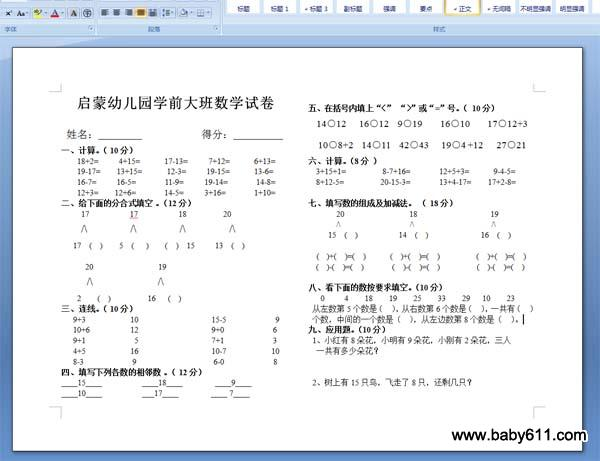# 启蒙幼儿园学前大班数学试卷启蒙幼儿园学前大班数学试卷姓名：                   得分：

一、计算。（ 10分）18+2=     4+15=      17-13=     7+12=      6+13=19-17=    13+15=      12-3=     19-15=      13-6=16-7=     16-5=       11-9=     19-14=       14-8=12+3=    12+6=       14-5=     3+16=       1+10=二、给下面的分合式填空 。（12分）17          17          18         20/\        /\        /\        /\17  (  )    5  (   )    (  )  15     13  (  )20                19/\            /\2    (  )         16   (  )三、连线。（ 10分）9+3           10                  15-5            910+6          12                  9+0            69+1            5                  7+1            34+5           16                  10-7           108-3            9                   6-0            8四、填写下列各数的相邻数 。（ 12分）____15____          ____18____        ____9________10____          ___17____        ____ 7____五、在括号内填上"〈" "〉"或"="号。（ 10分）14○12   16○12  9○19    16○10    17○12+310○8+2  14○11  42○43   19○4 +12   27○21六、计算。（8分 ）3+15+1=         8-7+16=       12+5+3=      9-4-5=8+12-5=         20-15-3=       13+4-17=    17+2-8=七、填写数的组成及加减法。 （ 18分）20                18                  19/\                 /\                   /\15  (  )          14  (  )             16  (  )(  )+(  )=(  )      (  )+(  )=(  )         (  )+(  )=(  )(  )-(  )=(  )       (  )-(  )=(  )         (  )-(  )=(  )八、看下面的数按要求填空。（10分）0     4    18    19    25    33    29   10    23从左数第5个数是（  ），从右数第6个数是（  ），一共有（  ）个数，中间的一个数是（  ），从左边数第8个数是（  ）。

• 幼儿园试卷分类
• 将此试卷分享到：
• 数学试卷最新更新